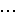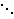# QR DecompositionSuppose we have a problem that can be modeled by the system of equations Ax = b with a matrix A and a vector b. We have already shown how to use Gaussian Elimination to solve these methods, but I would like to introduce you to another method – QR decomposition. In addition to solving the general system of equations, this method can also be used in a number of other algorithms including the linear regression analysis to solve the least squares problem and to find the eigenvalues of a matrix.

Generally, when we see a system of equations, the best way to proceed in solving it is Gaussian elimination, or LU decomposition. However, there are some very special matrices where this method can lead to rounding errors. In such cases, we need a better, or more stable algorithm, which is when the QR decomposition method becomes important.

Any matrix A, of dimensions m by n with m >= n, can be represented as the product of two matrices, an m by m orthogonal matrix Q, i.e. QTQ = I, and an m by n upper triangular matrix R with the form [R 0]T. We perform this decomposition by will first converting the columns of the matrix A into vectors. Then, for each vector ak, we will calculate the vectors uk and ek given by

uk =i = 1 to k-1projej ak
and
ek = uk / ||uk||
Then Q = [e1, …, en] and

R =00 0# Learn About “the Other” AlgebraWhen I visit family for the holidays, the topic of my being a mathematician always seems to come up, and there’s always a child in the family struggling with maths, and when I ask the subject of their struggles the word “algebra” is always the culprit. I’ll save for another post my ideas on how this subject should be taught in high school and some of the main problems facing students.

I want to concentrate this post on a topic that few outside the mathematical world know about, but which many inside this world (myself included) hold dearly – the topic of modern or abstract algebra. I refer to this as “the other” algebra because a general conversation about the word “algebra” will generally revolve around concepts such as systems of equations, slopes, intercepts, intersection, rise-over-run, point-slope, and other terminology that limits algebra to a specific domain (the set of real or complex numbers) while at the same time ignoring the underlying beauty associated with this area.

I wrote previously about the area of set theory and the beauty associated with taking math out of the scope of a basic number line and into a much more undefined space. Abstract algebra is a continuation of set theory where in addition to our set, we have a (binary) operation defined on any two elements of this set. The inclusion of this binary operation allows us to consider several different structures based on the properties that this binary operation holds.

The structures I’d like to write about today are called groups. A group is a set along with an operation (or function) defined on any two elements of the set with the following properties:
– It is closed. This means that any time we run this function on two elements on the set, the function gives us a member of the set. In mathematical terms, for all a, b in the set A, f(a, b) must also be a member of A.
– There is an identity element. An identity element is defined as an element where is we include it in the binary operator with any other element, the operator will always return the other element. So if the element i is the identity element, then f(i, a) = a and f(a, i) = a for any other a in the set A. Any group must have an identity element.
– Every element has an inverse. Inverse elements are based on the identity element. What the inverse says is that for every element, there is a way to use the binary operator to get to the identity element. So for all elements a in the set A, there is an element b in the set A such that f(a, b) = i, where i is the identity element.
– The binary operator is associative. I described the associative property when I discussed the functions and relations of set theory. A function is associative if the way we group things (aka associate them) doesn’t matter. This means that for any elements a, b, and c of the set A, f(f(a, b), c) must be the same as f(a, f(b, c)).

If these four properties hold for a set A and a binary function f, then we say that the pair (A, f) is a group. We will generally use a common notation such as a · b, or a * b or simply ab to represent f(a, b).

Another important concept in group theory is the idea of a Cayley table. These are similar to multiplication tables that we drew out when we were first learning our “times tables”. For a group with n elements, we form a table with n rows and n columns. Each element of the group is written out to the left of each row and above each column (so really we can think of it as an n+1 by n+1 table with the first row and column being descriptive rows). Each cell of the table is the binary operator applied to the two elements indicated by the row and column (with an understanding of whether we have row before column or vice versa). Obviously, we can only do this for finite groups as we cannot write out all the elements of an infinite set.

The script I’ve added is a tester to allow users to input the information for a possible group (size, name of each element, and a Cayley table) and with this information the user is informed whether or not it forms a group. If it does not form a group, the reasons why it does not form one are also given. There are also some sample groups given to give insight into this area.